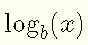# Convert Logarithms and Exponentials

## Relationship Between Exponential and Logarithm

The logarithmic functionsand the exponential functionsare inverse of each other, hencewhere $$b$$ is the common base of the exponential and the logarithm.
The above equivalence helps in solving logarithmic and exponential functions and needs a deep understanding. Examples, of how the above relationship between the logarithm and exponential may be used to transform expressions and solve problems are presented below.

### Example 1

Change each logarithmic expression to an exponential expression.
1. $$\log_3 27 = 3$$
2. $$\log_{36} 6 = 1 / 2$$
3. $$\log_2 (1 / 8) = -3$$
4. $$\log_8 2 = 1 / 3$$

Solution to Example 1:
1. The logarithmic form $$\log_3 27 = 3$$ is equivalent to the exponential form $27 = 3^3$ 2. The logarithmic expression $$\log_{36} 6 = 1 / 2$$ is equivalent to the exponential expression $6 = 36^{1/2}$ 3. The expression $$\log_2 (1 / 8) = - 3$$ in exponential form is given by $1 / 8 = 2^{-3}$ 4. $$\log_8 2 = 1 / 3$$ in exponential form is given by $2 = 8^{1/3}$

### Example 2

Change each exponential expression to a logarithmic expression.
1. $$3^4 = 81$$
2. $$4^{1/2} = 2$$
3. $$3^{-1/3} = 1 / 27$$
4. $$10^3 = 1000$$

Solution to Example 2:
1. The exponential form $$3^4 = 81$$ is equivalent to the logarithmic form $4 = \log_3 (81)$ 2. The exponential form $$4^{1/2} = 2$$ is equivalent to the logarithmic form $1 / 2 = \log_4 2$ 3. $$3^{-3} = 1 / 27$$ in logarithmic form is given by $-3 = \log_3 (1/ 27)$ 4. $$10^3 = 1000$$ in logarithmic form is given by $3 = \log_{10} 1000$

### Example 3

Solve for $$x$$ the following equations.
1. $$\log_3 x = 5$$
2. $$\log_2 (x - 3) = 2$$
3. $$2 \log_3 (- x + 1) = 6$$

Solution to Example 3:
1. To solve the equation $$\log_3 x = 5$$, rewrite it into exponential form $x = 3^5$ 2. Rewrite the equation $$\log_2 (x - 3) = 2$$ into exponential form $x - 3 = 2^2 = 4$ Solve for x $x = 4 + 3 = 7$ 3.Divide all terms of the equation $$2 \log_3 (- x + 1) = 6$$ by 2 $\log_3 (- x + 1) = 3$ Rewrite the equation obtained in exponential form $- x + 1 = 3^3 = 27$ Solve for x $x = - 26$